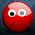## Binary Search Tree (BST) Algorithm Tutorial

Earlier we had a tutorial on Binary Seach Tree Basics, which you can check for refreshing the knowledge about it. Today we will be taking a look on BST algorithm and implementing it using Java.

Binary Search Tree is node based binary tree data structure with the following properties:

* The Left subtree contains the nodes with keys less than the node's key.
* The Right subtree contains the nodes with keys greater than the node's key.
* Both the right and left subtree should also be binary search tree.
* There should not be any duplicate nodes.

We have implemented below operations of Binary Search Tree:

* Searching
* Insert Node
* MinValue
* MaxValue

We will be seeing each of the operation and the corresponding Java code.

But before we go on and look at the operations. Here is the Node.java class that we will be using.

```package BST;

public class Node {

protected Node left;
protected Node right;
protected int value;

public Node(int value){
this.value=value;
}
}

```

SEARCHING

Searching can be a recursive or iterative process.

We begin first by comparing the value with the root node and if the value is equal to root node value then the search is successful. Otherwise we check if it is greater or smaller than root node. If smaller we go to the left subtree and repeat the above process and if its greater we go to the right subtree and repeat the above process.

This operation of searching requires O(log n) time.

Pseudo Code:

search for a matching node
1. Start at the root node as current node
2. If the search key’s value matches the current node’s key then found a match
3. If search key’s value is greater than current node’s
1. If the current node has a right child, search right
2. Else, no matching node in the tree
4. If search key is less than the current node’s
1. If the current node has a left child, search left
2. Else, no matching node in the tree

Java Implementation:

```    public void search(Node n, int value){
if(n.value == value || n==null){
System.out.println("\nFound Value: " + n.value);
}else if(value<n.value){
search(n.left,value);
}else {
search(n.right,value);
}
}

```

Insert Node

Insert Node operation also behaves in the same manner as Searching operation. Firstly, it checks whether the key is the same as that of root, if not then we either choose the right subtree or the left subtree depending upon the value passed is greater or smaller than the root node value respectively.

Eventually, we will reach an external node where we will add the new node as its left or right child depending upon the node's key.

This is also a recursive operation, as we start from the root and go until we find the right place to insert to node.

It takes O(log n) time i.e. proportional to the height of the tree.

Pseudo Code:

Always insert new node as leaf node
2. Start at root node as current node
3. If new node’s key < current’s key
1. If current node has a left child, search left
2. Else add new node as current’s left child
4. If new node’s key > current’s key
1. If current node has a right child, search right
2. Else add new node as current’s right child

Java Code:

```    public void insert(Node n, int value){
if(value < n.value){
if(n.left != null){
insert(n.left,value);
}else{
n.left=new Node(value);
}
}
if(value > n.value){
if(n.right != null){
insert(n.right,value);
}else{
n.right=new Node(value);
}
}
}
```

MinValue and MaxValue

In this operation we find he minimum and maximum value in the tree.

it also take O(log n) time.

Java Code:

```    public int minValue(Node n){
while(n.left!=null){
n=n.left;
}
return n.value;
}

public int maxValue(Node n){
while(n.right!=null){
n=n.right;
}
return n.value;
}

```

The next tutorial will be on BST Traversal algorithms and their Java implementation.

1.This post is very useful for us. Because we have a lot of
tips and tricks from this post. Thank you for this amazing post share. I many
2.3.